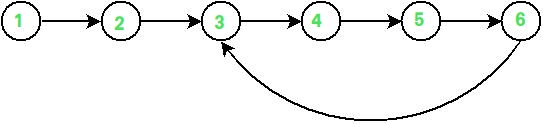Related Articles

# Find duplicates in constant array with elements 0 to N-1 in O(1) space

• Difficulty Level : Hard
• Last Updated : 03 May, 2021

Given a constant array of n elements which contains elements from 1 to n-1, with any of these numbers appearing any number of times. Find any one of these repeating numbers in O(n) and using only constant memory space.
Examples:

```Input : arr[] = {1, 2, 3, 4, 5, 6, 3}
Output : 3```

As the given array is constant methods given in below articles will not work.

So, here is an approach that is based on Floyd’s cycle finding algorithm. We use this to detect loops in a linked list.
The idea is to consider array items as linked list nodes. Any particular index is pointing to the value at that index. And you will see that there is a loop as shown in the image below- In case of a duplicate, two indexes will have same value, and they will form a cycle just like in the image given below.
Linked list formed for above example would be :
1->2->3->4->5->6->3So we can find the entry point of cycle in the linked list and that will be our duplicate element.

1. We maintain two pointers fast and slow
2. For each step fast will move to the index that is equal to arr[arr[fast]](two jumps at a time) and slow will move to the index arr[slow](one step at a time)
3. When fast==slow that means now we are in a cycle.
4. Fast and slow will meet in a circle and the entry point of that circle will be the duplicate element.
5. Now we need to find entry point so we will start with fast=0 and visit one step at a time for both fast and slow.
6. When fast==slow that will be entry point.
7. Return the entry point.

## C++

 `// CPP program to find a duplicate``// element in an array with values in``// range from 0 to n-1.``#include ``using` `namespace` `std;` `// function to find one duplicate``int` `findduplicate(``const` `int` `arr[], ``int` `n)``{``    ``// return -1 because in these cases``    ``// there can not be any repeated element``    ``if` `(n <= 1)``        ``return` `-1;` `    ``// initialize fast and slow``    ``int` `slow = arr;``    ``int` `fast = arr[arr];` `    ``// loop to enter in the cycle``    ``while` `(fast != slow) {` `        ``// move one step for slow``        ``slow = arr[slow];` `        ``// move two step for fast``        ``fast = arr[arr[fast]];``    ``}` `    ``// loop to find entry point of the cycle``    ``fast = 0;``    ``while` `(slow != fast) {``        ``slow = arr[slow];``        ``fast = arr[fast];``    ``}``    ``return` `slow;``}` `int` `main()``{``    ``const` `int` `arr[] = { 1, 2, 3, 4, 5, 6, 3 };``    ``int` `n = ``sizeof``(arr) / ``sizeof``(arr);``    ``cout << findduplicate(arr, n);``    ``return` `0;``}`

## Java

 `// Java program to find a duplicate``// element in an array with values in``// range from 0 to n-1.``import` `java.io.*;``import` `java.util.*;` `public` `class` `GFG {``     ` `    ``// function to find one duplicate``    ``static` `int` `findduplicate(``int` `[]arr, ``int` `n)``    ``{``         ` `        ``// return -1 because in these cases``        ``// there can not be any repeated element``        ``if` `(n <= ``1``)``            ``return` `-``1``;``     ` `        ``// initialize fast and slow``        ``int` `slow = arr[``0``];``        ``int` `fast = arr[arr[``0``]];``     ` `        ``// loop to enter in the cycle``        ``while` `(fast != slow)``        ``{``     ` `            ``// move one step for slow``            ``slow = arr[slow];``     ` `            ``// move two step for fast``            ``fast = arr[arr[fast]];``        ``}``     ` `        ``// loop to find entry``        ``// point of the cycle``        ``fast = ``0``;``        ``while` `(slow != fast)``        ``{``            ``slow = arr[slow];``            ``fast = arr[fast];``        ``}``        ``return` `slow;``    ``}``     ` `    ``// Driver Code``    ``public` `static` `void` `main(String args[])``    ``{``        ``int` `[]arr = {``1``, ``2``, ``3``, ``4``, ``5``, ``6``, ``3``};``        ``int` `n = arr.length;``        ``System.out.print(findduplicate(arr, n));``    ``}``}`` ` `// This code is contributed by``// Manish Shaw (manishshaw1)`

## Python 3

 `# Python 3 program to find a duplicate``# element in an array with values in``# range from 0 to n-1.` `# function to find one duplicate``def` `findduplicate(arr, n):` `    ``# return -1 because in these cases``    ``# there can not be any repeated element``    ``if` `(n <``=` `1``):``        ``return` `-``1` `    ``# initialize fast and slow``    ``slow ``=` `arr[``0``]``    ``fast ``=` `arr[arr[``0``]]` `    ``# loop to enter in the cycle``    ``while` `(fast !``=` `slow) :` `        ``# move one step for slow``        ``slow ``=` `arr[slow]` `        ``# move two step for fast``        ``fast ``=` `arr[arr[fast]]` `    ``# loop to find entry point of the cycle``    ``fast ``=` `0``    ``while` `(slow !``=` `fast):``        ``slow ``=` `arr[slow]``        ``fast ``=` `arr[fast]``    ``return` `slow` `# Driver Code``if` `__name__ ``=``=` `"__main__"``:``    ` `    ``arr ``=` `[``1``, ``2``, ``3``, ``4``, ``5``, ``6``, ``3` `]``    ``n ``=` `len``(arr)``    ``print``(findduplicate(arr, n))` `# This code is contributed by ita_c`

## C#

 `// C# program to find a duplicate``// element in an array with values in``// range from 0 to n-1.``using` `System;``using` `System.Collections.Generic;``class` `GFG {``    ` `    ``// function to find one duplicate``    ``static` `int` `findduplicate(``int` `[]arr, ``int` `n)``    ``{``        ` `        ``// return -1 because in these cases``        ``// there can not be any repeated element``        ``if` `(n <= 1)``            ``return` `-1;``    ` `        ``// initialize fast and slow``        ``int` `slow = arr;``        ``int` `fast = arr[arr];``    ` `        ``// loop to enter in the cycle``        ``while` `(fast != slow)``        ``{``    ` `            ``// move one step for slow``            ``slow = arr[slow];``    ` `            ``// move two step for fast``            ``fast = arr[arr[fast]];``        ``}``    ` `        ``// loop to find entry``        ``// point of the cycle``        ``fast = 0;``        ``while` `(slow != fast)``        ``{``            ``slow = arr[slow];``            ``fast = arr[fast];``        ``}``        ``return` `slow;``    ``}``    ` `    ``// Driver Code``    ``public` `static` `void` `Main()``    ``{``        ``int` `[]arr = {1, 2, 3, 4, 5, 6, 3};``        ``int` `n = arr.Length;``        ``Console.Write(findduplicate(arr, n));``    ``}``}` `// This code is contributed by``// Manish Shaw (manishshaw1)`

## PHP

 ``

## Javascript

 ``

Output:

`3`

Time Complexity : O(n)
Auxiliary Space : O(1)

Attention reader! Don’t stop learning now. Get hold of all the important DSA concepts with the DSA Self Paced Course at a student-friendly price and become industry ready.  To complete your preparation from learning a language to DS Algo and many more,  please refer Complete Interview Preparation Course.

In case you wish to attend live classes with experts, please refer DSA Live Classes for Working Professionals and Competitive Programming Live for Students.

My Personal Notes arrow_drop_up